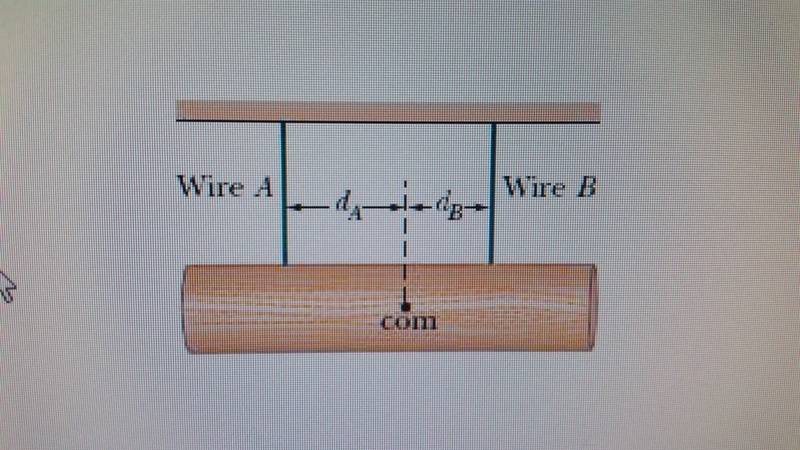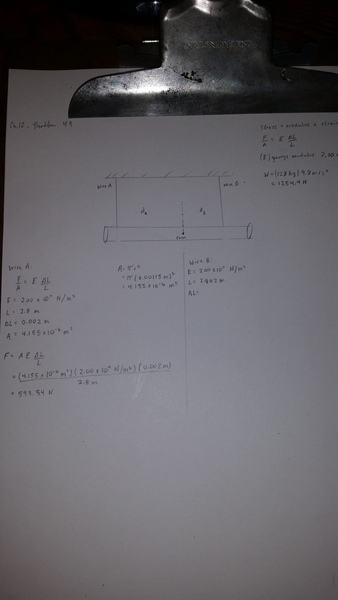# Log hanging from 2 steel wires. stress/strain youngs modulus

## Homework Statement

In the figure, a 128 kg uniform log hangs by two steel wires, A and B, both of radius 1.15 mm. Initially, wire A was 2.80 m long and 2.00 mm shorter than wire B. The log is now horizontal. Young's modulus for steel is 2.00 × 1011 N/m2.

What are the magnitudes of the forces on it from (a) wire A and (b) wire B? (c) What is the ratio dA/dB?## Homework Equations

$$Force/Area = E (ΔL/L)$$
E=2.00x1011N/m2
L is length of wire

## The Attempt at a Solutionedit. i managed to get the forces, working on da/db

Last edited:

does this look ok for FA?
$$W/2 + dAE/2L$$
W is weight of log
d is .002m
A is area of wire
E young's modulus
L length of wire.

SteamKing
Staff Emeritus
Homework Helper
does this look ok for FA?
$$W/2 + dAE/2L$$
W is weight of log
d is .002m
A is area of wire
E young's modulus
L length of wire.
Only if you assume that the wires have the weight of the log evenly distributed between them, which doesn't seem to be the case, given the problem statement.

Go back to first principles here. Write the equations of static equilibrium which govern the log.

Pay careful attention to what the problem is telling you about the lengths of the wires:

a. Wire A is 2.80 m long and was 2 mm shorter than wire B initially, which I take to mean before the log was attached.

b. After the log has been attached, the shorter wire A and the longer wire B are now both the same length, which is why the log is horizontal. This suggests that wire A has stretched more than wire B, which also implies that the force acting in wire A is greater than the force acting in wire B, after the log is attached.

c. If you figure out the ratio of the forces in wires A and B which make them the same length, then you can also figure out the location of the wires relative to the c.o.m. of the log, because you have written the equations of static equilibrium for the log and the wires.

c. If you figure out the ratio of the forces in wires A and B which make them the same length, then you can also figure out the location of the wires relative to the c.o.m. of the log, because you have written the equations of static equilibrium for the log and the wires.

Im not sure how the forces are related to distance outside of finding the moment. M=Fd.
FA/FB ≠ dA/dB

this is what i have so far;
$$-FAdA+FBdB=0$$

i still need another question though

Last edited:
TSny
Homework Helper
Gold Member
Looks like you assumed that wire A will stretch 2 mm. But wire B is also going to stretch some. So wire A will need to stretch more than 2 mm in order for the final length of A to equal the final length of B.

[Edit: I now see that you mentioned that you have found the forces. I think you have the right idea that you will need to consider moments in order to get the ratio of the distances.]

Looks like you assumed that wire A will stretch 2 mm. But wire B is also going to stretch some. So wire A will need to stretch more than 2 mm in order for the final length of A to equal the final length of B.
Yea i happen to assumed correctly based on a sample problem in the book. Im stuck on the last part its asking for dA/dB

FA=924N
FB=330.4N

TSny
Homework Helper
Gold Member
Im not sure how the forces are related to distance outside of finding the moment. M=Fd.
FA/FB ≠ dA/dB

this is what i have so far;
$$-FAdA+FBdB=0$$

Can you solve this for the ratio of d's?

•J-dizzal
SteamKing
Staff Emeritus
Homework Helper
Im not sure how the forces are related to distance outside of finding the moment. M=Fd.
FA/FB ≠ dA/dB

this is what i have so far;
$$-FAdA+FBdB=0$$

i still need another question though
It's not clear what you mean by needing "another question".

You won't be able to see how the ratio of the forces is related to the location of the c.o.m. relative to the wires unless you write the equations of statics, especially the moment equation.

It's not complicated. You can do it in a minute or so.

It's not clear what you mean by needing "another question".

You won't be able to see how the ratio of the forces is related to the location of the c.o.m. relative to the wires unless you write the equations of statics, especially the moment equation.

It's not complicated. You can do it in a minute or so.

I only needed one equation which is the moment equation, yes it had two unknowns but they are solved as a ratio. I didnt realize this at first but know i do. thank you.